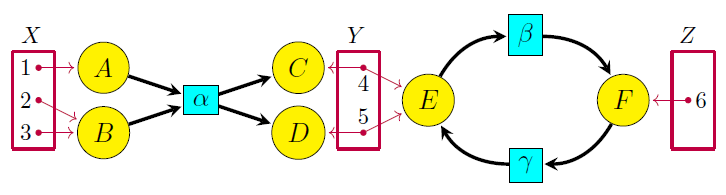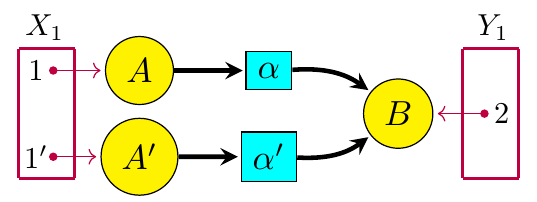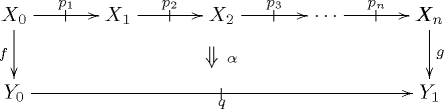## Open Petri Nets (Part 1)

Jade Master and I have just finished a paper on open Petri nets:

• John Baez and Jade Master, Open Petri nets, Mathematical Structures in Computer Science, 30 (2020), 314–341.

Abstract. The reachability semantics for Petri nets can be studied using open Petri nets. For us an ‘open’ Petri net is one with certain places designated as inputs and outputs via a cospan of sets. We can compose open Petri nets by gluing the outputs of one to the inputs of another. Open Petri nets can be treated as morphisms of a category, which becomes symmetric monoidal under disjoint union. However, since the composite of open Petri nets is defined only up to isomorphism, it is better to treat them as morphisms of a symmetric monoidal double category$\mathbb{O}\mathbf{pen}(\mathrm{Petri}).$ Various choices of semantics for open Petri nets can be described using symmetric monoidal double functors out of$\mathbb{O}\mathbf{pen}(\mathrm{Petri}).$ Here we describe the reachability semantics, which assigns to each open Petri net the relation saying which markings of the outputs can be obtained from a given marking of the inputs via a sequence of transitions. We show this semantics gives a symmetric monoidal lax double functor from$\mathbb{O}\mathbf{pen}(\mathrm{Petri})$ to the double category of relations. A key step in the proof is to treat Petri nets as presentations of symmetric monoidal categories; for this we use the work of Meseguer, Montanari, Sassone and others.

I’m excited about this, especially because our friends at Statebox are planning to use open Petri nets in their software. They’ve recently come out with a paper too:

• Fabrizio Romano Genovese and Jelle Herold, Executions in (semi-)integer Petri nets are compact closed categories.

Petri nets are widely used to model open systems in subjects ranging from computer science to chemistry. There are various kinds of Petri net, and various ways to make them ‘open’, and my paper with Jade only handles the simplest. But our techniques are flexible, so they can be generalized.

What’s an open Petri net? For us, it’s a thing like this:

The yellow circles are called ‘places’ (or in chemistry, ‘species’). The aqua rectangles are called ‘transitions’ (or in chemistry, ‘reactions’). There can in general be lots of places and lots of transitions. The bold arrows from places to transitions and from transitions to places complete the structure of a Petri net. There are also arbitrary functions from sets$X$ and$Y$ into the set of places. This makes our Petri net into an ‘open’ Petri net.

We can think of open Petri nets as morphisms between finite sets. There’s a way to compose them! Suppose we have an open Petri net$P$ from$X$ to$Y,$ where now I’ve given names to the points in these sets:We write this as$P \colon X \nrightarrow Y$ for short, where the funky arrow reminds us this isn’t a function between sets. Given another open Petri net$Q \colon Y \nrightarrow Z,$ for example this:the first step in composing$P$ and$Q$ is to put the pictures together:At this point, if we ignore the sets$X,Y,Z,$ we have a new Petri net whose set of places is the disjoint union of those for$P$ and$Q.$

The second step is to identify a place of$P$ with a place of$Q$ whenever both are images of the same point in$Y$. We can then stop drawing everything involving$Y,$ and get an open Petri net$QP \colon X \nrightarrow Z,$ which looks like this:Formalizing this simple construction leads us into a bit of higher category theory. The process of taking the disjoint union of two sets of places and then quotienting by an equivalence relation is a pushout. Pushouts are defined only up to canonical isomorphism: for example, the place labeled$C$ in the last diagram above could equally well have been labeled$D$ or$E.$ This is why to get a category, with composition strictly associative, we need to use isomorphism classes of open Petri nets as morphisms. But there are advantages to avoiding this and working with open Petri nets themselves. Basically, it’s better to work with things than mere isomorphism classes of things! If we do this, we obtain not a category but a bicategory with open Petri nets as morphisms.

However, this bicategory is equipped with more structure. Besides composing open Petri nets, we can also ‘tensor’ them via disjoint union: this describes Petri nets being run in parallel rather than in series. The result is a symmetric monoidal bicategory. Unfortunately, the axioms for a symmetric monoidal bicategory are cumbersome to check directly. Double categories turn out to be more convenient.

Double categories were introduced in the 1960s by Charles Ehresmann. More recently they have found their way into applied mathematics. They been used to study various things, including open dynamical systems:

• Eugene Lerman and David Spivak, An algebra of open continuous time dynamical systems and networks.

open electrical circuits and chemical reaction networks:

• Kenny Courser, A bicategory of decorated cospans, Theory and Applications of Categories 32 (2017), 995–1027.

open discrete-time Markov chains:

• Florence Clerc, Harrison Humphrey and P. Panangaden, Bicategories of Markov processes, in Models, Algorithms, Logics and Tools, Lecture Notes in Computer Science 10460, Springer, Berlin, 2017, pp. 112–124.

and coarse-graining for open continuous-time Markov chains:

• John Baez and Kenny Courser, Coarse-graining open Markov processes. (Blog article here.)

As noted by Shulman, the easiest way to get a symmetric monoidal bicategory is often to first construct a symmetric monoidal double category:

• Mike Shulman, Constructing symmetric monoidal bicategories.

The theory of ‘structured cospans’ gives a systematic way to build symmetric monoidal double categories—Kenny Courser and I are writing a paper on this—and Jade and I use this to construct the symmetric monoidal double category of open Petri nets.

A 2-morphism in a double category can be drawn as a square like this:We call$X_1,X_2,Y_1$ and$Y_2$ ‘objects’,$f$ and$g$ ‘vertical 1-morphisms’,$M$ and$N$ ‘horizontal 1-cells’, and$\alpha$ a ‘2-morphism’. We can compose vertical 1-morphisms to get new vertical 1-morphisms and compose horizontal 1-cells to get new horizontal 1-cells. We can compose the 2-morphisms in two ways: horizontally and vertically. (This is just a quick sketch of the ideas, not the full definition.)

In our paper, Jade and I start by constructing a symmetric monoidal double category$\mathbb{O}\mathbf{pen}(\textrm{Petri})$ with:

• sets$X, Y, Z, \dots$ as objects,

• functions$f \colon X \to Y$ as vertical 1-morphisms,

• open Petri nets$P \colon X \nrightarrow Y$ as horizontal 1-cells,

• morphisms between open Petri nets as 2-morphisms.

(Since composition of horizontal 1-cells is associative only up to an invertible 2-morphism, this is technically a pseudo double category.)

What are the morphisms between open Petri nets like? A simple example may be help give a feel for this. There is a morphism from this open Petri net:to this one:mapping both primed and unprimed symbols to unprimed ones. This describes a process of ‘simplifying’ an open Petri net. There are also morphisms that include simple open Petri nets in more complicated ones, etc.

This is just the start. Our real goal is to study the semantics of open Petri nets: that is, how they actually describe processes! And for that, we need to think about the free symmetric monoidal category on a Petri net. You can read more about those things in Part 2 and Part 3 of this series.

Part 1: the double category of open Petri nets.

Part 2: the reachability semantics for open Petri nets.

Part 3: the free symmetric monoidal category on a Petri net.

### 13 Responses to Open Petri Nets (Part 1)

1.Joe Moeller says:

Typo: “What are the morphisms between open Petri nets ilke?”

•John Baez says:

You and your ilke!Thanks.

2.Eugene says:

Symmetric monoidal categories give rise to representable multicategories. What do monoidal double categories give rise to?

Also, algebras over multicategories give semantics (I think).
What do algebras over monoidal double categories give you?

•John Baez says:

Eugene wrote:

Symmetric monoidal categories give rise to representable multicategories. What do monoidal double categories give rise to?

I don’t know! This concept bubbles to the surface of my mind:

• nLab, Virtual double categories.

I’m not claiming this concept is the answer to your question – it’s certainly not.

But it’s still an interesting concept. The basic sort of 2-cell in a virtual double category looks like this:so virtual double categories are a common generalization of monoidal category, bicategory, double category, and multicategory!

Also, algebras over multicategories give semantics (I think). What do algebras over monoidal double categories give you?

More semantics. ‘Semantics’ is a very general term for ‘mapping syntactic expressions to their meanings’. Lawvere’s thesis Functorial Semantics showed how to do semantics using maps between categories with finite products. Later people generalized this to all kinds of categories, and in our paper we’re doing it with symmetric monoidal double categories.

•Eugene says:

I saw your paper in the arxiv last night, so that kind of answers my question about semantics in more details. I guess I was too fixated on operads.

I think I have an idea of what a double multicategory should be like, and I have a live example or two, which are mentioned in arXiv:1705.04814 [math.OC]. But maybe I am wrong.

3.Simon Willerton says:

Does each of your vertical 1-morphisms, ie. function between sets, have a conjoint and companion open Petri net? In other words, do you have a fibrant double category (or an equipment, if you prefer)?

•John Baez says:

Yes!

This is why I said Mike Shulman’s technique can be applied to get a symmetric monoidal bicategory from the symmetric monoidal double category of open Petri nets. His technique applies to fibrant double categories—or more generally, ‘isofibrant’ ones, where each invertible vertical 1-morphism has a conjoint and a companion.

Jade and I don’t actually discuss this isofibrancy, or how to get the symmetric monoidal bicategory. Kenny Courser and I will talk about these things more generally in our paper on structured cospans. The double category$\mathbb{O}\mathbf{pen}(\mathrm{Petri})$ is an example of a ‘structured cospan double category’. The idea is that an open Petri net is a cospan of sets where the apex is equipped with extra structure: it’s made into the set of places of a Petri net. Structured cospan double categories are isofibrant under very general conditions!

• John Baez and Jade Master, Open Petri nets.

Last time I explained, in a sketchy way, the double category of open Petri nets. This time I’d like to describe a ‘semantics’ for open Petri nets.

5. […] Open Petri Nets (Part 1) 4 by gbrown_ | 0 comments on Hacker News. […]

6.Seth Buehler says:

Not sure if this is the right place for it, but in page 20 of your paper, the proof of theorem 18 specifies that$R_1$ and$R_2$ are /linear/ relations, but everything in sight is only a set, not an Abelian group, so I don’t even know what that would mean.

•John Baez says:

Thanks for catching that! Just delete the world “linear”. We took a proof for the double category of linear relations between vector spaces from my paper with Kenny Courser, and adapted it… but failed to purge it of all linearity!

7. If$L \colon \mathsf{A} \to \mathsf{X}$ is the functor from sets to Petri nets sending each set to the Petri having that set of places and nothing else, a structured cospan looks like this:

• John Baez, Open Petri nets, Azimuth, 15 August 2018.

8. This talk on structured cospans and Petri nets is the second of a two-part series, but it should be understandable on its own. The first part is on structured cospans and double categories.

You can see my talk live on YouTube here, with simultaneous discussion on the Category Theory Community Server. (To join this, click here.) The talk will be recorded and remain available on YouTube.

You can already see the slides here.

Structured cospans and Petri nets

Abstract. “Structured cospans” are a general way to study networks with inputs and outputs. Here we illustrate this using a type of network popular in theoretical computer science: Petri nets. An “open” Petri net is one with certain places designated as inputs and outputs. We can compose open Petri nets by gluing the outputs of one to the inputs of another. Using the formalism of structured cospans, open Petri nets can be treated as morphisms of a symmetric monoidal category—or better, a symmetric monoidal double category. We explain two forms of semantics for open Petri nets using symmetric monoidal double functors out of this double category. The first, an operational semantics, gives for each open Petri net a category whose morphisms are the processes that this net can carry out. The second, a “reachability” semantics, simply says what these processes can accomplish. This is joint work with Kenny Courser and Jade Master.

This site uses Akismet to reduce spam. Learn how your comment data is processed.